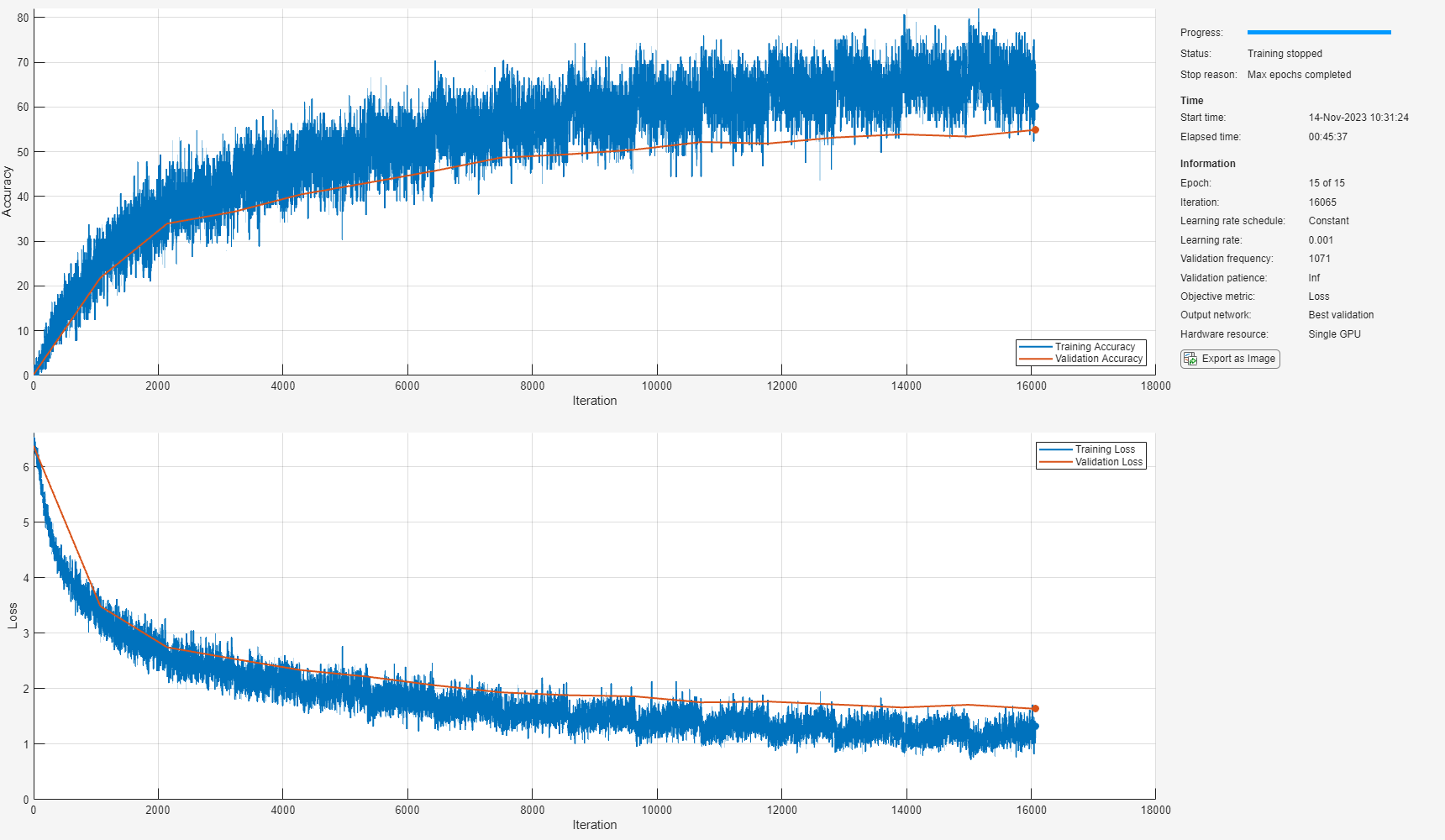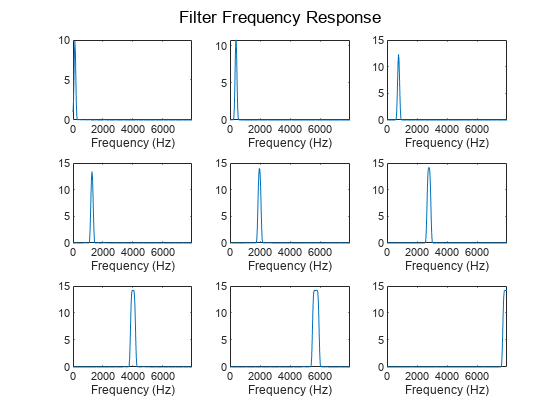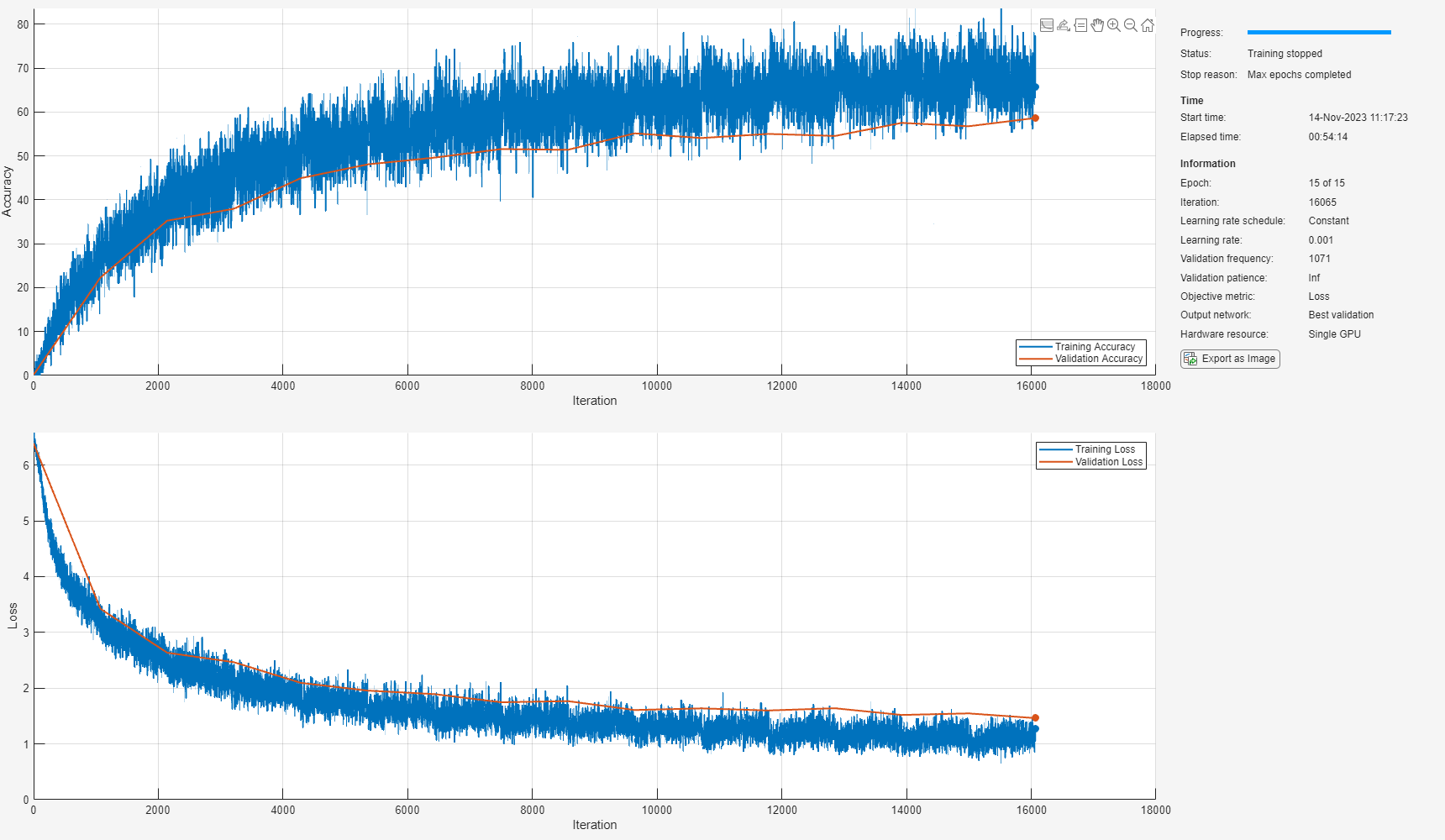Main Content

# Speaker Identification Using Custom SincNet Layer and Deep Learning

In this example, you train three convolutional neural networks (CNNs) to perform speaker verification and then compare the performances of the architectures. The architectures of the three CNNs are all equivalent except for the first convolutional layer in each:

1. In the first architecture, the first convolutional layer is a "standard" convolutional layer, implemented using `convolution2dLayer`.

2. In the second architecture, the first convolutional layer is a constant sinc filterbank, implemented using a custom layer.

3. In the third architecture, the first convolutional layer is a trainable sinc filterbank, implemented using a custom layer. This architecture is referred to as SincNet .

 shows that replacing the standard convolutional layer with a filterbank layer leads to faster training convergence and higher accuracy.  also shows that making the parameters of the filter bank learnable yields additional performance gains.

### Introduction

Speaker identification is a prominent research area with a variety of applications including forensics and biometric authentication. Many speaker identification systems depend on precomputed features such as i-vectors or MFCCs, which are then fed into machine learning or deep learning networks for classification. Other deep learning speech systems bypass the feature extraction stage and feed the audio signal directly to the network. In such end-to-end systems, the network directly learns low-level audio signal characteristics.

In this example, you first train a traditional end-to-end speaker identification CNN. The filters learned tend to have random shapes that do not correspond to perceptual evidence or knowledge of how the human ear works, especially in scenarios where the amount of training data is limited . You then replace the first convolutional layer in the network with a custom sinc filterbank layer that introduces structure and constraints based on perceptual evidence. Finally, you train the SincNet architecture, which adds learnability to the sinc filterbank parameters.

The three neural network architectures explored in the example are summarized as follows:

1. Standard Convolutional Neural Network - The input waveform is directly connected to a randomly initialized convolutional layer which attempts to learn features and capture characteristics from the raw audio frames.

2. ConstantSincLayer - The input waveform is convolved with a set of fixed-width sinc functions (bandpass filters) equally spaced on the mel scale.

3. SincNetLayer - The input waveform is convolved with a set of sinc functions whose parameters are learned by the network. In the SincNet architecture, the network tunes parameters of the sinc functions while training.

This example defines and trains the three neural networks proposed above and evaluates their performance on the LibriSpeech Dataset .

### Data Set

#### Download Dataset

In this example, you use a subset of the LibriSpeech Dataset . The LibriSpeech Dataset is a large corpus of read English speech sampled at 16 kHz. The data is derived from audiobooks read from the LibriVox project.

```downloadDatasetFolder = tempdir; filename = "train-clean-100.tar.gz"; url = "http://www.openSLR.org/resources/12/" + filename; datasetFolder = fullfile(downloadDatasetFolder,"LibriSpeech","train-clean-100"); if ~isfolder(datasetFolder) gunzip(url,downloadDatasetFolder); unzippedFile = fullfile(downloadDatasetFolder,filename); untar(unzippedFile{1}(1:end-3),downloadDatasetFolder); end```

Create an `audioDatastore` object to access the LibriSpeech audio data.

`ADS = audioDatastore(datasetFolder,'IncludeSubfolders',1);`

Extract the speaker label from the file path.

`ADS.Labels = extractBetween(ADS.Files,fullfile(datasetFolder,filesep),filesep);`

The full `dev-train-100` dataset is around 6 GB of data. To train the network with data from all 251 speakers, set `reduceDataset` to `false`. To run this example quickly with data from just six speakers, set `reduceDataset` to `true`.

```reducedDataSet =false; if reducedDataSet indices = cellfun(@(c)str2double(c)<50,ADS.Labels); %#ok ADS = subset(ADS,indices); end ADS = splitEachLabel(ADS,0.1);```

Split the audio files into training and test data. 80% of the audio files are assigned to the training set and 20% are assigned to the test set.

`[ADSTrain,ADSTest] = splitEachLabel(ADS,0.8);`

#### Sample Speech Signal

Plot one of the audio files and listen to it.

```[audioIn,dsInfo] = read(ADSTrain); Fs = dsInfo.SampleRate; sound(audioIn,Fs) t = (1/Fs)*(0:length(audioIn)-1); plot(t,audioIn) title("Audio Sample") xlabel("Time (s)") ylabel("Amplitude") grid on```Reset the training datastore.

`reset(ADSTrain)`

#### Data Preprocessing

CNNs expect inputs to have consistent dimensions. You will preprocess the audio by removing regions of silence and then break the remaining speech into 200 ms frames with 40 ms overlap.

Set the parameters for preprocessing.

```frameDuration = 200e-3; overlapDuration = 40e-3; frameLength = floor(Fs*frameDuration); overlapLength = round(Fs*overlapDuration);```

Use the supporting function, preprocessAudioData`, `to preprocess the training and test data. `XTrain` and `XTest` contain the train and test speech frames, respectively. `YTrain` and `YTest` contain the train and test labels, respectively.

`[XTrain,YTrain] = preprocessAudioData(ADSTrain,frameLength,overlapLength,Fs);`
```Starting parallel pool (parpool) using the 'local' profile ... Connected to the parallel pool (number of workers: 6). ```
`[XTest,YTest] = preprocessAudioData(ADSTest,frameLength,overlapLength,Fs);`

### Standard CNN

#### Define Layers

The standard CNN is inspired by the neural network architecture in .

```numFilters = 80; filterLength = 251; numSpeakers = numel(unique(ADS.Labels)); layers = [ imageInputLayer([1 frameLength 1]) % First convolutional layer convolution2dLayer([1 filterLength],numFilters) batchNormalizationLayer leakyReluLayer(0.2) maxPooling2dLayer([1 3]) % This layer is followed by 2 convolutional layers convolution2dLayer([1 5],60) batchNormalizationLayer leakyReluLayer(0.2) maxPooling2dLayer([1 3]) convolution2dLayer([1 5],60) batchNormalizationLayer leakyReluLayer(0.2) maxPooling2dLayer([1 3]) % This is followed by 3 fully-connected layers fullyConnectedLayer(256) batchNormalizationLayer leakyReluLayer(0.2) fullyConnectedLayer(256) batchNormalizationLayer leakyReluLayer(0.2) fullyConnectedLayer(256) batchNormalizationLayer leakyReluLayer(0.2) fullyConnectedLayer(numSpeakers) softmaxLayer classificationLayer];```

Analyze the layers of the neural network using the `analyzeNetwork` function

`analyzeNetwork(layers)`

#### Train Network

Train the neural network for 15 epochs using `adam` optimization. Shuffle the training data before every epoch. The training options for the neural network are set using `trainingOptions`. Use the test data as the validation data to observe how the network performance improves as training progresses.

```numEpochs = 15; miniBatchSize = 128; validationFrequency = floor(numel(YTrain)/miniBatchSize); options = trainingOptions("adam", ... "Shuffle","every-epoch", ... "MiniBatchSize",miniBatchSize, ... "Plots","training-progress", ... "Verbose",false,"MaxEpochs",numEpochs, ... "ValidationData",{XTest,categorical(YTest)}, ... "ValidationFrequency",validationFrequency);```

To train the network, call `trainNetwork`.

`[convNet,convNetInfo] = trainNetwork(XTrain,YTrain,layers,options);`#### Inspect Frequency Response of First Convolutional Layer

Plot the magnitude frequency response of nine filters learned from the standard CNN network. The shape of these filters is not intuitive and does not correspond to perceptual knowledge. The next section explores the effect of using constrained filter shapes.

```F = squeeze(convNet.Layers(2,1).Weights); H = zeros(size(F)); Freq = zeros(size(F)); for ii = 1:size(F,2) [h,f] = freqz(F(:,ii),1,251,Fs); H(:,ii) = abs(h); Freq(:,ii) = f; end idx = linspace(1,size(F,2),9); idx = round(idx); figure for jj = 1:9 subplot(3,3,jj) plot(Freq(:,idx(jj)),H(:,idx(jj))) sgtitle("Frequency Response of Learned Standard CNN Filters") xlabel("Frequency (Hz)") end```### Constant Sinc Filterbank

In this section, you replace the first convolutional layer in the standard CNN with a constant sinc filterbank layer. The constant sinc filterbank layer convolves the input frames with a bank of fixed bandpass filters. The bandpass filters are a linear combination of two sinc filters in the time domain. The frequencies of the bandpass filters are spaced linearly on the mel scale.

#### Define Layers

The implementation for the constant sinc filterbank layer can be found in the `constantSincLayer.m` file (attached to this example). Define parameters for a `ConstantSincLayer`. Use 80 filters and a filter length of 251.

```numFilters = 80; filterLength = 251; numChannels = 1; name = 'constant_sinc';```

Change the first convolutional layer from the standard CNN to the `ConstantSincLayer` and keep the other layers unchanged.

`cSL = constantSincLayer(numFilters,filterLength,Fs,numChannels,name)`
```cSL = constantSincLayer with properties: Name: 'constant_sinc' NumFilters: 80 SampleRate: 16000 FilterLength: 251 NumChannels: [] Filters: [1×251×1×80 single] MinimumFrequency: 50 MinimumBandwidth: 50 StartFrequencies: [1×80 double] Bandwidths: [1×80 double] Show all properties ```
`layers(2) = cSL;`

#### Train Network

Train the network using the `trainNetwork` function. Use the same training options defined previously.

`[constSincNet,constSincInfo] = trainNetwork(XTrain,YTrain,layers,options);`#### Inspect Frequency Response of First Convolutional Layer

The `plotNFilters` method plots the magnitude frequency response of `n` filters with equally spaced filter indices. Plot the magnitude frequency response of nine filters in the `ConstantSincLayer`.

```figure n = 9; plotNFilters(constSincNet.Layers(2),n)```### SincNet

In this section, you use a trainable SincNet layer as the first convolutional layer in your network. The SincNet layer convolves the input frames with a bank of bandpass filters. The bandwidth and the initial frequencies of the SincNet filters are initialized as equally spaced in the mel scale. The SincNet layer attempts to learn better parameters for these bandpass filters within the neural network framework.

#### Define Layers

The implementation for the SincNet layer filterbank layer can be found in the `sincNetLayer.m` file (attached to this example). Define parameters for a `SincNetLayer`. Use 80 filters and a filter length of 251.

```numFilters = 80; filterLength = 251; numChannels = 1; name = 'sinc';```

Replace the `ConstantSincLayer` from the previous network with the `SincNetLayer`. This new layer has two learnable parameters: `FilterFrequencies` and `FilterBandwidths`.

`sNL = sincNetLayer(numFilters,filterLength,Fs,numChannels,name)`
```sNL = sincNetLayer with properties: Name: 'sinc' NumFilters: 80 SampleRate: 16000 FilterLength: 251 NumChannels: [] Window: [1×251 double] TimeStamps: [1×251 double] MinimumFrequency: 50 MinimumBandwidth: 50 Learnable Parameters FilterFrequencies: [1×80 double] FilterBandwidths: [1×80 double] Show all properties ```
`layers(2) = sNL;`

#### Train Network

Train the network using the `trainNetwork` function. Use the same training options defined previously.

`[sincNet,sincNetInfo] = trainNetwork(XTrain,YTrain,layers,options);`#### Inspect Frequency Response of First Convolutional Layer

Use the `plotNFilters` method of `SincNetLayer` to visualize the magnitude frequency response of nine filters with equally spaced indices learned by SincNet.

```figure plotNFilters(sincNet.Layers(2),9)```### Results Summary

#### Accuracy

The table summarizes the frame accuracy for all three neural networks.

```NetworkType = {'Standard CNN','Constant Sinc Layer','SincNet Layer'}'; Accuracy = [convNetInfo.FinalValidationAccuracy;constSincInfo.FinalValidationAccuracy;sincNetInfo.FinalValidationAccuracy]; resultsSummary = table(NetworkType,Accuracy)```
```resultsSummary=3×2 table NetworkType Accuracy _______________________ ________ {'Standard CNN' } 72.97 {'Constant Sinc Layer'} 74.902 {'SincNet Layer' } 78.062 ```

#### Performance with Respect to Epochs

Plot the accuracy on the test set against the epoch number to see how well the networks learn as the number of epochs increase. SincNet outperforms the `ConstantSincLayer` network, especially during the early stages of training. This shows that updating the parameters of the bandpass filters within the neural network framework leads to faster convergence. This behavior is only observed when the dataset is large enough, so it might not be seen when `reduceDataSet` is set to `true.`

```epoch = linspace(0,numEpochs,numel(sincNetInfo.ValidationAccuracy(~isnan(sincNetInfo.ValidationAccuracy)))); epoch = [epoch,numEpochs]; sinc_valAcc = [sincNetInfo.ValidationAccuracy(~isnan(sincNetInfo.ValidationAccuracy)),... sincNetInfo.FinalValidationAccuracy]; const_sinc_valAcc = [constSincInfo.ValidationAccuracy(~isnan(constSincInfo.ValidationAccuracy)),... constSincInfo.FinalValidationAccuracy]; conv_valAcc = [convNetInfo.ValidationAccuracy(~isnan(convNetInfo.ValidationAccuracy)),... convNetInfo.FinalValidationAccuracy]; figure plot(epoch,sinc_valAcc,'-*','MarkerSize',4) hold on plot(epoch,const_sinc_valAcc,'-*','MarkerSize',4) plot(epoch,conv_valAcc,'-*','MarkerSize',4) ylabel('Frame-Level Accuracy (Test Set)') xlabel('Epoch') xlim([0 numEpochs+0.3]) title('Frame-Level Accuracy Versus Epoch') legend("sincNet","constantSincLayer","conv2dLayer","Location","southeast") grid on```In the figure above, the final frame accuracy is a bit different from the frame accuracy that is computed in the last iteration. While training, the batch normalization layers perform normalization over mini-batches. However, at the end of training, the batch normalization layers normalize over the entire training data, which results in a slight change in performance.

### Supporting Functions

```function [X,Y] = preprocessAudioData(ADS,SL,OL,Fs) if ~isempty(ver('parallel')) pool = gcp; numPar = numpartitions(ADS,pool); else numPar = 1; end parfor ii = 1:numPar X = zeros(1,SL,1,0); Y = zeros(0); subADS = partition(ADS,numPar,ii); while hasdata(subADS) [audioIn,dsInfo] = read(subADS); speechIdx = detectSpeech(audioIn,Fs); numChunks = size(speechIdx,1); audioData = zeros(1,SL,1,0); for chunk = 1:numChunks % Remove trail end audio audio_chunk = audioIn(speechIdx(chunk,1):speechIdx(chunk,2)); audio_chunk = buffer(audio_chunk,SL,OL); q = size(audio_chunk,2); % Split audio into 200 ms chunks audio_chunk = reshape(audio_chunk,1,SL,1,q); % Concatenate with existing audio audioData = cat(4,audioData,audio_chunk); end audioLabel = str2double(dsInfo.Label{1}); % Generate labels for training and testing by replecating matrix audioLabelsTrain = repmat(audioLabel,1,size(audioData,4)); % Add data points for current speaker to existing data X = cat(4,X,audioData); Y = cat(2,Y,audioLabelsTrain); end XC{ii} = X; YC{ii} = Y; end X = cat(4,XC{:}); Y = cat(2,YC{:}); Y = categorical(Y); end```

### References

 M. Ravanelli and Y. Bengio, "Speaker Recognition from Raw Waveform with SincNet," 2018 IEEE Spoken Language Technology Workshop (SLT), Athens, Greece, 2018, pp. 1021-1028, doi: 10.1109/SLT.2018.8639585.

 V. Panayotov, G. Chen, D. Povey and S. Khudanpur, "Librispeech: An ASR corpus based on public domain audio books," 2015 IEEE International Conference on Acoustics, Speech and Signal Processing (ICASSP), Brisbane, QLD, 2015, pp. 5206-5210, doi: 10.1109/ICASSP.2015.7178964

## SupportGet trial now